DAY 20
0
AI & Data

在seq2seq之前

RNN Encoder-Decoder

Google 正式提出 seq2seq 架構之前， Cho 等人就提出了以標準循環神經網絡（ standard RNN ）設計編碼器-解碼器的演算法：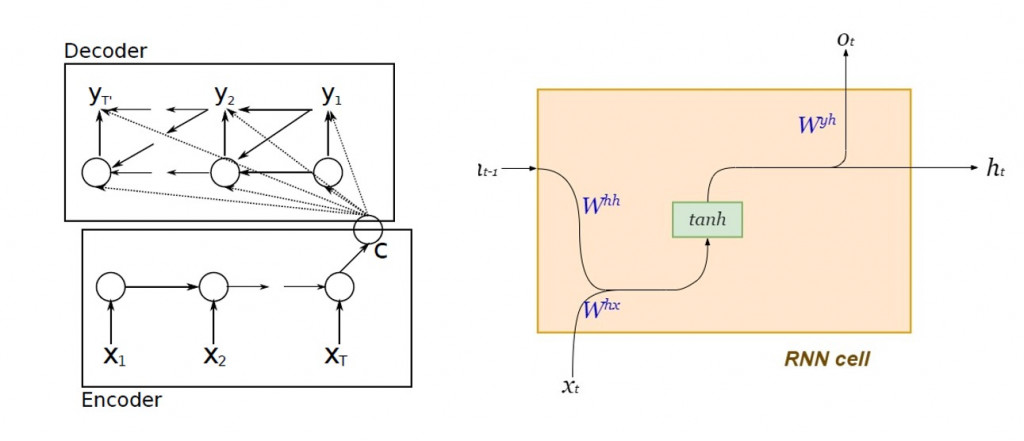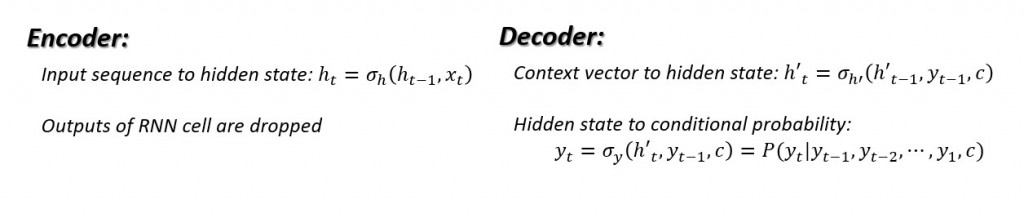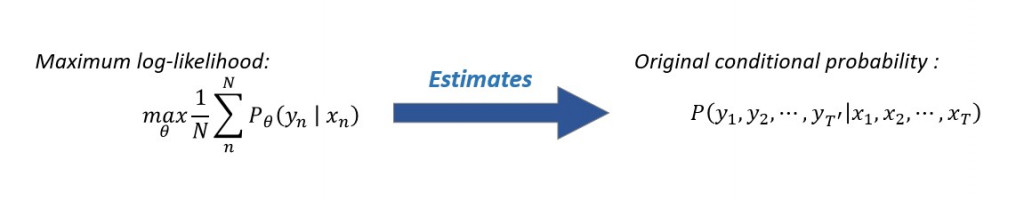單層LSTM Encoder-Decoder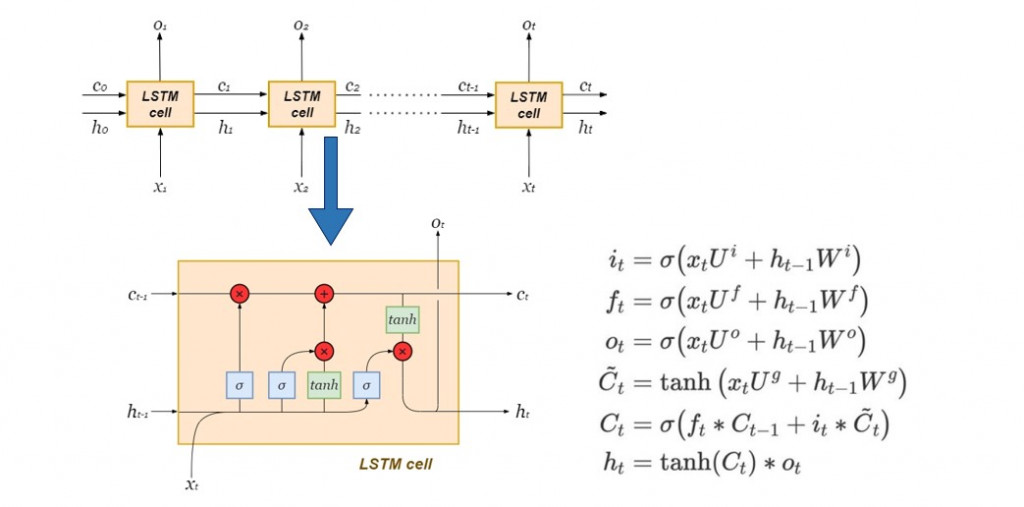Hidden state捕捉短期記憶，而cell state則儲存長期記憶：
Characterization is an abstract term that merely serves to illustrate how the hidden state is more concerned with the most recent time-step. The cell state is basically the global or aggregate memory of the LSTM network over all time-steps.

寫一個簡單的seq2seq網絡吧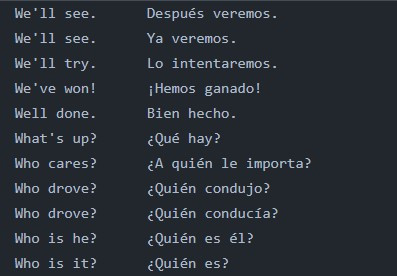資料準備

import numpy as np
from preprocess_text import input_docs, target_docs, input_tokens, target_tokens, num_encoder_tokens, num_decoder_tokens, max_encoder_seq_length, max_decoder_seq_length

encoder_input_data = np.zeros(
shape = (len(input_docs), max_encoder_seq_length, num_encoder_tokens),
dtype = "float32"
)

# Build out the decoder_input_data matrix:
decoder_input_data = np.zeros(
(len(input_docs), max_decoder_seq_length, num_decoder_tokens),
dtype = "float32"
)
# Build out the decoder_target_data matrix:
decoder_target_data = np.zeros(
(len(target_docs), max_decoder_seq_length, num_decoder_tokens),
dtype = "float32"
)

# since we're using a bilingual parallel corpus, we have the same number of sentences in both languages, therefore same size of input and target documents
for line, (input_doc, target_doc) in enumerate(zip(input_docs, target_docs)):
for timestep, token in enumerate(re.findall(r"[\w']+|[^\s\w]", input_doc)):
encoder_input_data[line, timestep, input_features_dict[token]] = 1.
for timestep, token in enumerate(target_doc.split()):
decoder_input_data[line, timestep, target_features_dict[token]] = 1.
if timestep > 0:
decoder_target_data[line, timestep - 1, target_features_dict[token]] = 1.